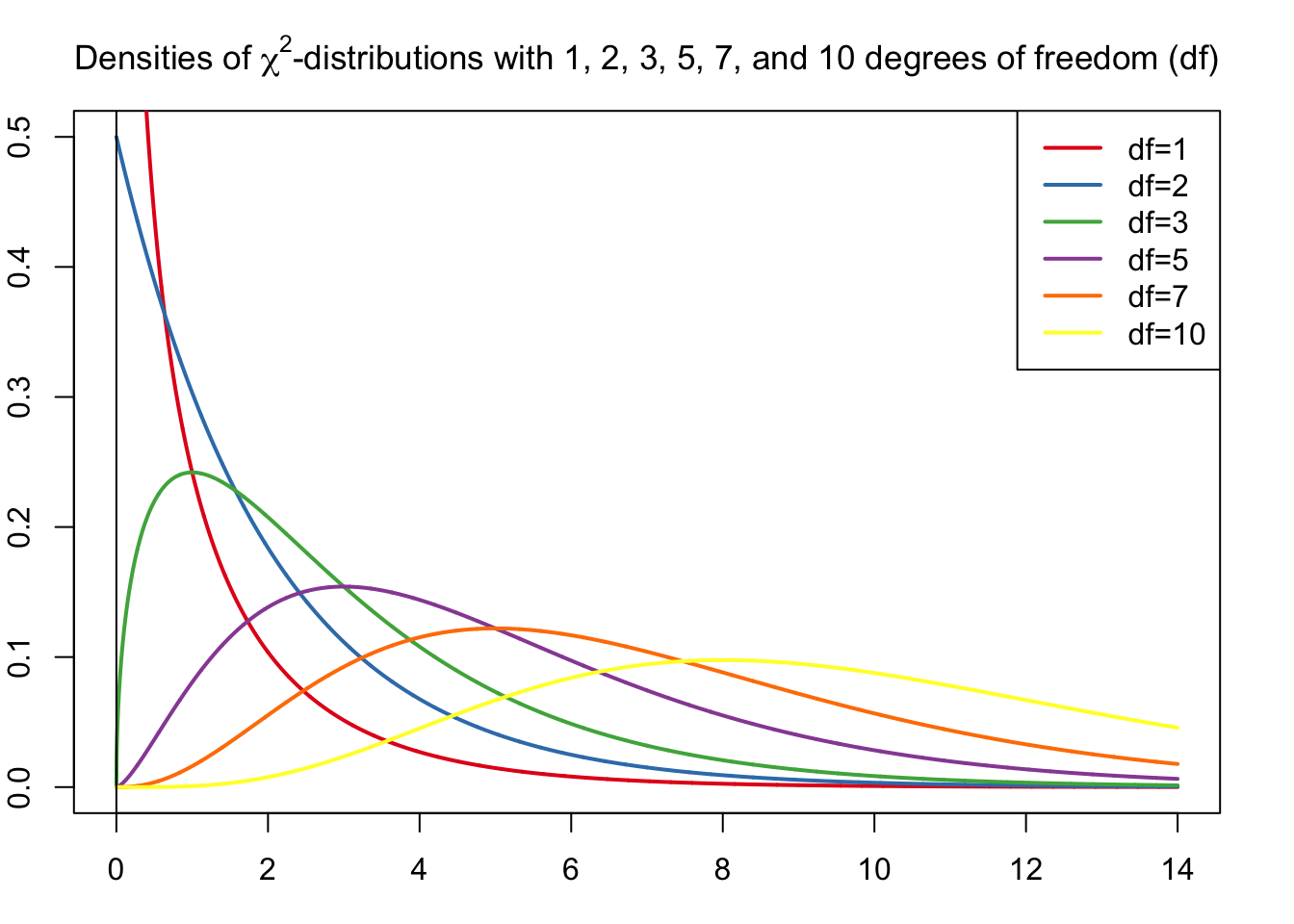The chi-square ($$\chi^2$$) distribution is one of the most important continuous probability distributions with many uses in statistical theory and inference (Lovric 2011).

Let $$n > 0$$ be a positive integer. For a random variable that has a ($$\chi^2$$)-distribution with $$n$$ degrees of freedom $$(df)$$ the probability density function is

$f(x) = \begin{cases} 0 & \text{if x \le 0} \\ \frac{x^{(n/2-1)}e^{-x/2}}{2^{n/2}\Gamma \left(\frac{k}{2}\right)} & \text{if x > 0} \end{cases}$

where $$\Gamma$$ denotes the Gamma function. The ($$\chi^2$$)-distribution (with $$n$$ degrees of freedom) is equal to the $$\Gamma$$-distribution with the parameters ($$n/2,2$$), that is, with the mean and variance equal to $$n$$ and $$2n$$ respectively.Basic Properties of $$\chi^2$$-Curves (Weiss 2010)

• The total area under a $$\chi^2$$-curve equals 1.
• A $$\chi^2$$-curve starts at 0 on the horizontal axis and extends indefinitely to the right, approaching, but never touching, the horizontal axis as it does so.
• A $$\chi^2$$-curve is right skewed.
• As the number of degrees of freedom becomes larger, $$\chi^2$$- curves look increasingly like normal curves.